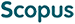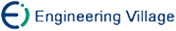罗四维 赵连伟. 基于谱图理论的流形学习算法[J]. 计算机研究与发展, 2006, 43(7): 1173-1179.
 引用本文: 罗四维 赵连伟. 基于谱图理论的流形学习算法[J]. 计算机研究与发展, 2006, 43(7): 1173-1179.Luo Siwei and Zhao Lianwei. Manifold Learning Algorithms Based on Spectral Graph Theory[J]. Journal of Computer Research and Development, 2006, 43(7): 1173-1179.
 Citation: Luo Siwei and Zhao Lianwei. Manifold Learning Algorithms Based on Spectral Graph Theory[J]. Journal of Computer Research and Development, 2006, 43(7): 1173-1179.## Manifold Learning Algorithms Based on Spectral Graph Theory

• 摘要: 流形学习的主要目标是发现嵌入在高维数据空间的低维光滑流形.近年来基于谱图理论的学习算法受到研究者的广泛关注.介绍了流形与流形学习的关系，着重研究了几种有代表性的基于谱图理论的流形学习算法，并对算法进行了比较分析，最后进行总结和对进一步的研究做了展望.

Abstract: In the problem of manifold learning, one seeks to find a smooth low-dimensional manifold embedded in the high-dimensional vector space, based on a set of sample points. Spectral graph theory studies the eigenvectors and eigenvalues of matrices associated with graphs and has been widely used in the manifold learning algorithm recently. In this paper, the relationship between the manifold and the manifold learning is introduced first, and then some typical manifold learning algorithms based on spectral graph theory are studied. Finally, some directions for further research are suggested./下载:  全尺寸图片 幻灯片
• 分享
• 用微信扫码二维码

分享至好友和朋友圈